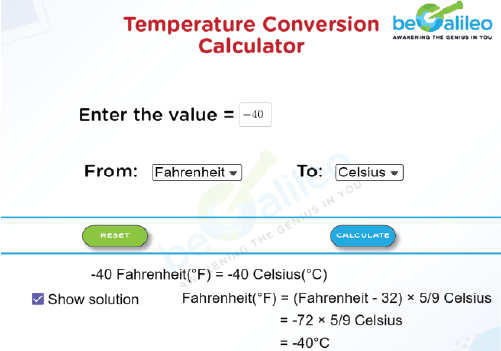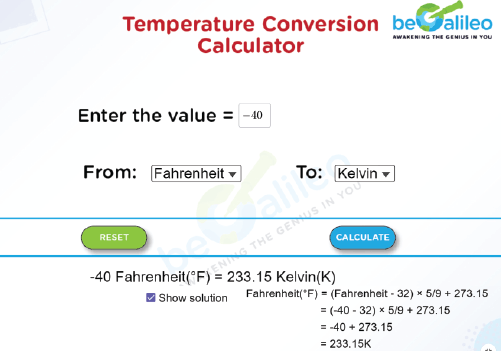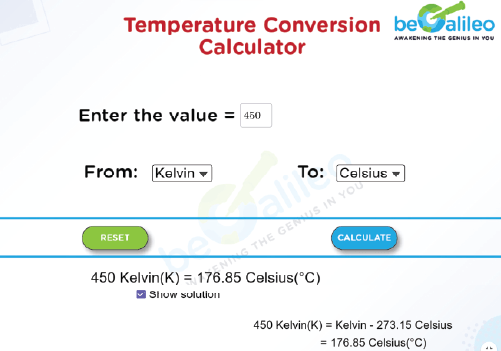>### Master Math with 1:1 Online Classes. Learn from Experts!# Temperature conversion calculator

A Temperature Conversion Calculator is a handy tool for changing temperatures between different systems. The main ones people use are Celsius (^\circ\text{C}) and Fahrenheit (^\circ\text{F}), but there's also Kelvin (K). People all over the world use these scales, and the converter makes it easy for scientists, engineers, weather experts, and others to switch between them.

Here's a brief overview of the most common temperature scales:

Celsius (^\circ\text{C}) : The Celsius scale defines its zero point as the freezing point of water, marked at 0^\circ\text{C}, and it's one-hundred-point as the boiling point of water, recorded as 100^\circ\text{C} (under standard atmospheric pressure). This scale enjoys global adoption, with the notable exception of the United States.

Fahrenheit (^\circ\text{F}) : The Fahrenheit scale, primarily utilized in the United States, designates the freezing point of water at 32^\circ\text{F} and the boiling point at 212^\circ\text{F} (under standard atmospheric pressure).

Kelvin (K) : The Kelvin scale stands as an absolute temperature measurement system, with 0 K signifying absolute zero, the point of lowest attainable temperature.

## How to use the Temperature Conversion Calculator?

Using a temperature conversion calculator is a straightforward process:

1. Enter the Value :
Input the temperature value you want to convert into the calculator.
2. Choose the Initial Scale(From) :
Start by selecting the temperature scale of the value you want to convert (e.g., Celsius, Fahrenheit, or Kelvin).
3. Select the Target Scale(To) :
Choose the scale you want to convert the temperature to (e.g., if you entered a Celsius temperature and want to convert it to Fahrenheit, select Fahrenheit as the target scale).
4. Click Calculate :
Press the ‘Calculate’ button to obtain the converted temperature.

If you want to view the calculation then click on the ‘Show Solution’ checkbox.

With these user-friendly steps, you can effortlessly perform temperature conversions and gain a deeper understanding of the conversion process if you choose to peek under the hood by revealing the solution.

## How to Convert Between the Temperature Scales?

Now, let's explore how to convert temperatures between these scales using a simple temperature converter formula:

1. Celsius to Fahrenheit :
Use the formula F=\left(\text{C} \times \frac{9}{5} \right) + 32
2. Fahrenheit to Celsius :
Use the formula C=(F - 32) \times \frac{5}{9}
3. Celsius to Kelvin :
Simply add 273.15 to the Celsius value to get Kelvin.
4. Kelvin to Celsius :
Subtract 273.15 from the Kelvin value to get Celsius.
5. Fahrenheit to Kelvin :
First, convert Fahrenheit to Celsius using the formula above, and then add 273.15 to the Celsius value.

Examples :

1. Convert 25 ^\circ\text{C} to Fahrenheit :
F = (25 \times \frac{9}{5}) + 32 =77^\circ\text{F}
2. Convert 98.6 ^\circ\text{F} to Celsius :
C =(98.6 - 32) \times \frac{5}{9} =37 ^\circ\text{C}
3. Convert -10 ^\circ\text{C} to Kelvin :
K = −10 + 273.15 = 263.15 K
4. Convert 300K to Celsius :
C = 300 − 273.15 = 26.85^\circ\text{C}

## Solved Examples

### Q1. If you have a temperature of -40^\circFahrenheit, what is it in both degrees Celsius and Kelvin?

Solution :
Converting -40^\circ Fahrenheit to Celsius
C = (-40 -32) \times \frac{5}{9} =-40 ^\circ\text{C}Converting -40^\circ Fahrenheit to Kelvin
Kelvin (K) = Celsius (^\circ\text{C}) + 273.15
= -40^\circ\text{C} + 273.15
= 233.15 K### Q2. You're studying a chemistry experiment, and the temperature is given as 450 Kelvin. Convert this temperature to degrees Celsius.

Solution :
To convert 450 Kelvin to degrees Celsius, you can use the formula:
Celsius (^\circ\text{C})= Kelvin (K) − 273.15
= 450 K − 273.15
= 176.85^\circ\text{C}